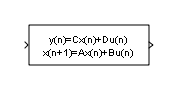Documentation

This is machine translation

Mouseover text to see original. Click the button below to return to the English version of the page.

Fixed-Point State-Space

Implement discrete-time state space

• Library:Description

The Fixed-Point State-Space block implements the system described by

`$y\left(n\right)=\text{C}x\left(n\right)+\text{D}u\left(n\right)$`
`$x\left(n+1\right)=\text{A}x\left(n\right)+\text{B}u\left(n\right)$`

where u is the input, x is the state, and y is the output. Both equations have the same data type.

• A must be an n-by-n matrix, where n is the number of states.

• B must be an n-by-m matrix, where m is the number of inputs.

• C must be an r-by-n matrix, where r is the number of outputs.

• D must be an r-by-m matrix.• The state x must be an n-by-1 vector.

• The input u must be an m-by-1 vector.

• The output y must be an r-by-1 vector.

The block accepts one input and generates one output. The width of the input vector is the number of columns in the B and D matrices. The width of the output vector is the number of rows in the C and D matrices. To define the initial state vector, use the Initial conditions parameter.

Ports

Input

expand all

Input vector, where the width equals the number of columns in the B and D matrices. For more information, see Description.

Data Types: `single` | `double` | `int8` | `int16` | `int32` | `uint8` | `uint16` | `uint32` | `Boolean` | `fixed point`

Output

expand all

Output signal, with width equal to the number of rows in the C and D matrices. For more information, see Description.

Data Types: `single` | `double` | `int8` | `int16` | `int32` | `uint8` | `uint16` | `uint32` | `Boolean` | `fixed point`

Parameters

expand all

Main

Specify the matrix of states as a real-valued n-by-n matrix, where n is the number of states. For more information on the matrix coefficients, see Description.

Programmatic Use

 Block Parameter: `A` Type: character vector Values: scalar | vector | matrix Default: ```'[2.6020 -2.2793 0.6708; 1 0 0; 0 1 0]'```

Specify the column vector of inputs as a real-valued n-by-m matrix, where n is the number of states, and m is the number of inputs. For more information on the matrix coefficients, see Description.

Programmatic Use

 Block Parameter: `B` Type: character vector Values: scalar | vector | matrix Default: `'[ 1; 0; 0]'`

Specify the column vector of outputs as a real-valued r-by-n matrix, where r is the number of outputs, and n is the number of states. For more information on the matrix coefficients, see Description.

Programmatic Use

 Block Parameter: `C` Type: character vector Values: scalar | vector | matrix Default: `'[0.0184 0.0024 0.0055]'`

Specify the matrix for direct feedthrough as a real-valued r-by-m matrix, where r is the number of outputs, and m is the number of inputs. For more information on the matrix coefficients, see Description.

Programmatic Use

 Block Parameter: `D` Type: character vector Values: scalar | vector | matrix Default: `'[0.0033]'`

Specify the initial condition for the state.

Limitations

The initial state cannot be `inf` or `NaN`.

Programmatic Use

 Block Parameter: `X0` Type: character vector Values: scalar | vector | matrix Default: `'0.0'`

Signal Attributes

Specify the data type the block uses for internal calculations.

Programmatic Use

 Block Parameter: `InternalDataType` Type: character vector Values: data type string Default: `'fixdt('double')'`

Specify the scaling for the state equation AX+BU.

Programmatic Use

 Block Parameter: `StateEqScaling` Type: character vector Values: scalar Default: `'2^0'`

Specify the scaling for the output equation CX+DU.

Programmatic Use

 Block Parameter: `InternalDataType` Type: character vector Values: scalar Default: `'2^0'`

Select to lock the output data type setting of this block against changes by the Fixed-Point Tool and the Fixed-Point Advisor. For more information, see Use Lock Output Data Type Setting (Fixed-Point Designer).

Programmatic Use

 Block Parameter: `LockScale` Type: character vector Values: `'off'` | `'on'` Default: `'off'`

Specify the rounding mode for fixed-point operations. For more information, see Rounding (Fixed-Point Designer).

Programmatic Use

 Block Parameter: `RndMeth` Type: character vector Values: ```'Ceiling' | 'Convergent' | 'Floor' | 'Nearest' | 'Round' | 'Simplest' | 'Zero'``` Default: `'Floor'`

When you select this check box, overflows saturate to the maximum or minimum value that the data type can represent. Otherwise, overflows wrap.

When you select this check box, saturation applies to every internal operation on the block, not just the output or result. In general, the code generation process can detect when overflow is not possible. In this case, the code generator does not produce saturation code.

Programmatic Use

 Block Parameter: `DoSatur` Type: character vector Values: `'off' | 'on'` Default: `'off'`

Block Characteristics

 Data Types `Boolean[a]` | `double` | `fixed point` | `integer` | `single` Direct Feedthrough `no` Multidimensional Signals `no` Variable-Size Signals `yes` Zero-Crossing Detection `no` [a] This block is not recommended for use with Boolean signals.